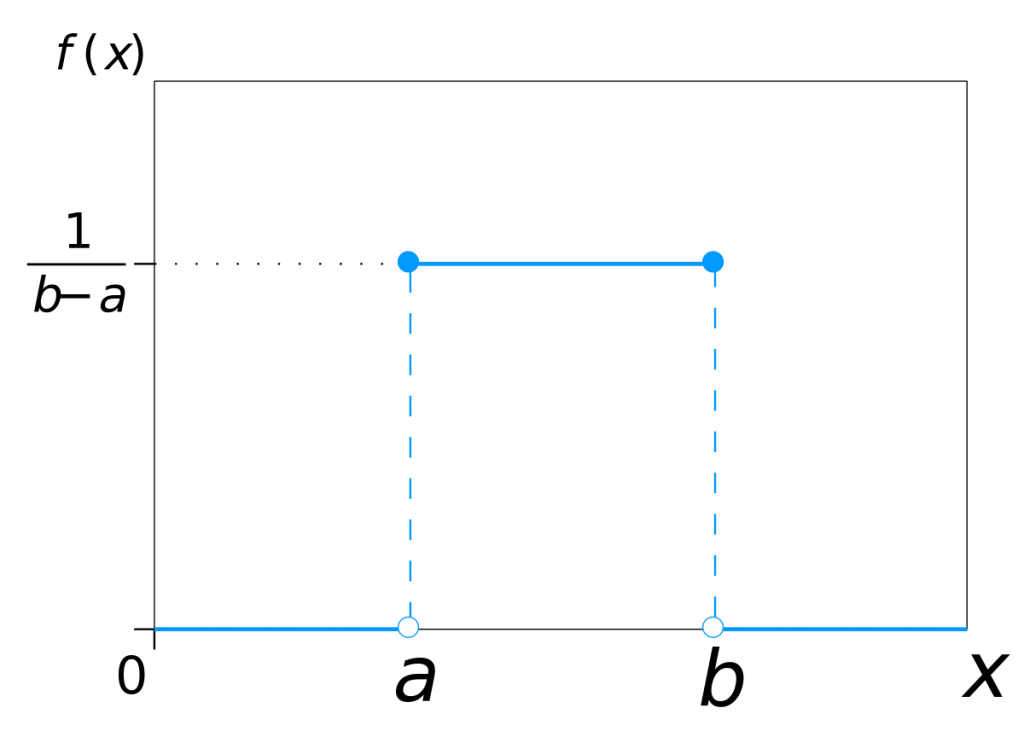# Uniform Probability DistributionIn a Uniform Distribution Probability Density Function (PDF) is same for all the possible X values. Sometimes this is called a Rectangular Distribution.

There are two (2) parameters in this distribution, a minimum (A) and a maximum (B)

It is written as U(A,B)

### Detailed Example

Waiting time for a bus is uniformly distributed between 0-15 minutes. We need to find the probability that a person waits less than 10 minutes.

We need to find the area of the shaded area to get the probability.

P(X ≤ 10) = 10 * (1/15) = 0.667